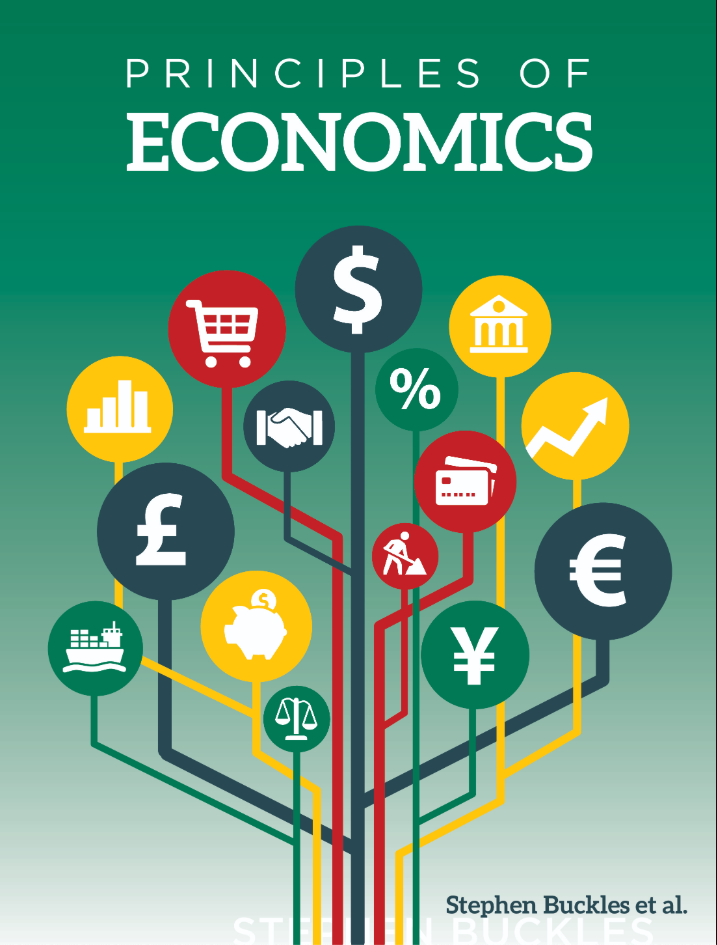# Principles of Economics

Principles of Economics will allow you to learn a new set of tools to use in personal, professional, business, and political decision making.

## What is a Top Hat Textbook?

Top Hat has reimagined the textbook – one that is designed to improve student readership through interactivity, is updated by a community of collaborating professors with the newest information, and accessed online from anywhere, at anytime.

• Top Hat Textbooks are built full of embedded videos, interactive timelines, charts, graphs, and video lessons from the authors themselves
• High-quality and affordable, at a significant fraction in cost vs traditional publisher textbooks

## Key features in this textbook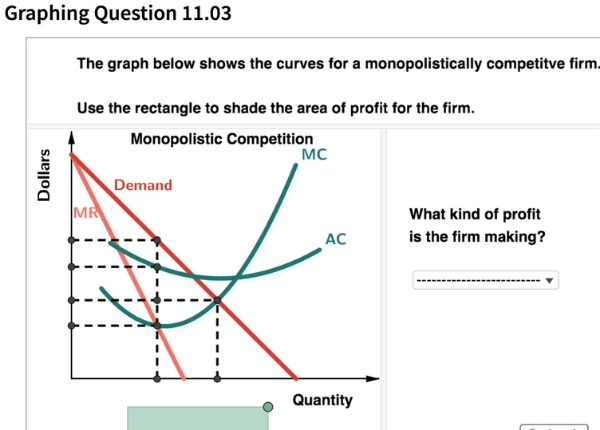Our Principles of Economics Textbooks extend beyond the page with interactive graphing tools, real-world news clips and articles that relate to current events and examples that are relevant to millennial audiences.

## Comparison of Principles of Economics Textbooks

Consider adding Top Hat’s Principles of Economics textbook to your upcoming course. We’ve put together a textbook comparison to make it easy for you in your upcoming evaluation.

### Top Hat

Stephen Buckles, Principles of Economics, Only One Edition needed

### Cengage

N. Gregory Mankiw, Principles of Economics, 8th Edition

### Pearson

Case, Fair, Oster, Principles of Economics, 12th Edition

### McGraw-Hill

McConnell, Brue, Flynn, Principles of Microeconomics, 7th Edition

### Pricing

Average price of textbook across most common format

#### $130 Hardcover print text only ####$175

Hardcover print text only

#### $140 Hardcover print text only ### Always up-to-date content, constantly revised by community of professors Content meets standard for Introduction to Anatomy & Physiology course, and is updated with the latest content ### In-Book Interactivity Includes embedded multi-media files and integrated software to enhance visual presentation of concepts directly in textbook Only available with supplementary resources at additional cost Only available with supplementary resources at additional cost Only available with supplementary resources at additional cost ### Customizable Ability to revise, adjust and adapt content to meet needs of course and instructor ### All-in-one Platform Access to additional questions, test banks, and slides available within one platform ## Pricing Average price of textbook across most common format ### Top Hat Stephen Buckles, Principles of Economics, Only One Edition needed #### Up to40-60%more affordable Lifetime access on any device ### Cengage N. Gregory Mankiw, Principles of Economics, 8th Edition ####$130

Hardcover print text only

### Pearson

Case, Fair, Oster, Principles of Economics, 12th Edition

#### $175 Hardcover print text only ### McGraw-Hill McConnell, Brue, Flynn, Principles of Microeconomics, 7th Edition ####$140

Hardcover print text only

## Always up-to-date content, constantly revised by community of professors

Constantly revised and updated by a community of professors with the latest content

### Top Hat

Stephen Buckles, Principles of Economics, Only One Edition needed

### Cengage

N. Gregory Mankiw, Principles of Economics, 8th Edition

### Pearson

Case, Fair, Oster, Principles of Economics, 12th Edition

### McGraw-Hill

McConnell, Brue, Flynn, Principles of Microeconomics, 7th Edition

## In-book Interactivity

Includes embedded multi-media files and integrated software to enhance visual presentation of concepts directly in textbook

### Top Hat

Stephen Buckles, Principles of Economics, Only One Edition needed

### Cengage

N. Gregory Mankiw, Principles of Economics, 8th Edition

### Pearson

Case, Fair, Oster, Principles of Economics, 12th Edition

### McGraw-Hill

McConnell, Brue, Flynn, Principles of Microeconomics, 7th Edition

## Customizable

Ability to revise, adjust and adapt content to meet needs of course and instructor

### Top Hat

Stephen Buckles, Principles of Economics, Only One Edition needed

### Cengage

N. Gregory Mankiw, Principles of Economics, 8th Edition

### Pearson

Case, Fair, Oster, Principles of Economics, 12th Edition

### McGraw-Hill

McConnell, Brue, Flynn, Principles of Microeconomics, 7th Edition

## All-in-one Platform

### Top Hat

Stephen Buckles, Principles of Economics, Only One Edition needed

### Cengage

N. Gregory Mankiw, Principles of Economics, 8th Edition

### Pearson

Case, Fair, Oster, Principles of Economics, 12th Edition

### McGraw-Hill

McConnell, Brue, Flynn, Principles of Microeconomics, 7th Edition

#### Stephen Buckles, Ph.DVanderbilt University

Stephen Buckles is a Senior Lecturer at Vanderbilt University, where he also received his Ph.D. in Economics. Buckles has been the recipient of numerous awards, including Madison Sarratt Prize for Excellence in Undergraduate Teaching (Vanderbilt, 2008), Kenneth G. Elzinga Distinguished Teaching Award (Southern Economic Association, 2006), and the Dean’s Award for Excellence in Teaching (Vanderbilt, 2007). His course pack, which this text is based on, has been used by thousands of students and engages the concepts of active learning.

#### PJ Glandon, PhDKenyon College

PJ Glandon joined Kenyon College as an Associate Professor of Economics after completing his Ph.D. at Vanderbilt University.

## Explore this textbook

Read the fully unlocked textbook below, and if you’re interested in learning more, get in touch to see how you can use this textbook in your course today.

# Chapter 7: Behind Supply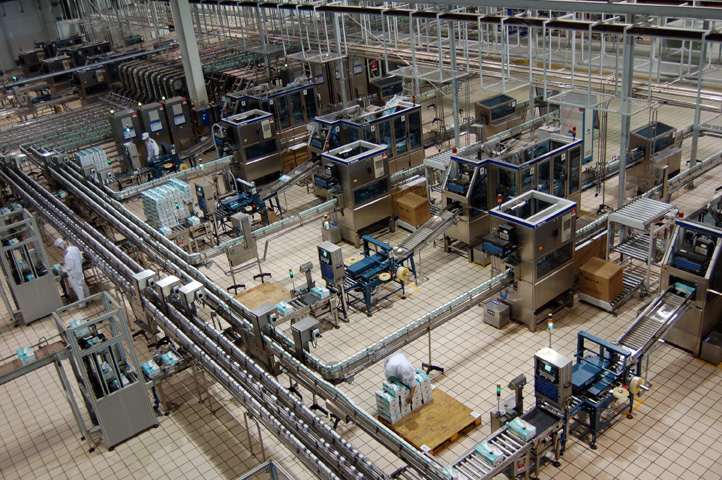Figure 7.1: You've got a factory; what happens when you add workers? To maximize profits, there is an optimal number of workers.​ ​

You have a summer internship with Patty’s Professional Pages (Patty’s Pages for short), a small web page development company. Patricia, your employer, gives you the task of evaluating a planned expansion in production. She asks you to determine how much the quantity produced will increase when new employees are hired. What will happen to her firm’s costs? Will it become more expensive or less expensive, on average, to produce new web pages? How will costs differ among alternative levels of output? The answers to those questions will prepare her for a decision as to the most profitable size for her business. If your advice is correct, she will offer you a permanent position after you graduate. If you are not helpful, she will buy you a ticket home.

## 7.1 Objectives

After reading this chapter and completing the questions and exercises, you will be able to:

• Explain why the rate of change in production varies as the amount of any single input used changes.
• Explain why total, average, and marginal costs change as the quantity produced increases or decreases.
• Construct graphs relating inputs to outputs and the level of output to various measures of cost.
• Identify the effects of changes in the productivity of variable inputs on production and cost functions.
• Identify the effects of changes in fixed and variable input prices on cost functions.

In Chapter Six, we explored the economic analysis that explains the shape and shifts of demand curves. In this and the next two chapters, we will develop the economic models that explain how producers make decisions about how much to produce – the supply decisions. We begin by developing a model of the production process, the relationship between inputs and output. We will first vary only one resource – the amount of labor. Then, we will expand the model by adding prices of inputs and discuss costs. In the next chapter, we will examine what happens when all inputs are changed. Finally, we will examine the type of market in which the producer competes and determine the market demand and supply as well as the equilibrium prices and quantities.

Think back to the discussion of the production possibilities frontier. Supply and demand direct the allocation of resources to specific products and determine where on the frontier an economy will be. As part of that process, those same forces guide individual producers to decide how much to produce.Figure 7.2: As an entrepreneur, Michael Dell has to make both short-run and long-run decisions.​ ​

Michael Dell is an entrepreneur. He developed a fairly simple idea that ultimately revolutionized the personal computer industry. His path from college student at the University of Texas at Austin to one of the world’s richest individuals highlights some of the important lessons of understanding the productivity of inputs.

While still a student, Mr. Dell earned money building and upgrading fellow students' computers with components available at local hobbyist shops. He recognized that the ability to make computers to order, which could be designed to consumers’ needs, had two advantages: He did not have to invest in a large warehouse to store finished computers waiting for buyers, and the consumers would get the computers that best met their needs. As his small project grew, he enlisted fellow students to help him expand output. When demand for computers outstripped the capacity of his dorm room, he invested in space in a business center and started hiring full-time employees. As his business grew, he built more factories and hired more workers. By the time Mr. Dell was 27, he had become the youngest CEO of a Fortune 500 company. Eventually, Mr. Dell invested in factory space in countries around the world.

We can view the growth of Dell Computers in light of the theme of this chapter. After each investment in new factory space, Mr. Dell was faced with the problem of how many workers to hire in that space. As production expanded, it would eventually be impractical to hire more workers in the space available. It would become necessary to look for more space.

## 7.2 Inputs and Outputs

Business owners normally have a number of goals, such as earning profits, providing employment in a community, building their firm’s share of the market, or developing a leading technology. In building a model that describes producer behavior, we will assume that a firm owner’s principal goal is to earn a profit – the difference between revenues and costs. In fact, many of the activities undertaken by firms can be reduced to profit-maximization; for instance, social philanthropy projects, such as donating a percentage of sales to a certain group, can be seen in light of increased market share as socially conscious consumers may choose to purchase their products, today and in the future, from that business.

When producers create goods and services to earn the revenue that generates their profits, they purchase raw materials and parts and unfinished goods. They purchase, rent, or hire what economists call factors of production labor, including all the physical and mental inputs of people; capital, including machines, buildings, tools, and inventories; and the land, which includes the raw materials from the land, water or air.

In our example of Patty’s Pages, before hiring you, Patricia rented office space and bought a certain number of computer workstations. She is in the process of determining how many employees are right for her shop. To do so, she will consider how the number of web pages designed each month changes as she changes the number of workers she hires. That relationship between the inputs – the size of the office, the amount of equipment, and the number of employees – and the number of web pages developed is Patty’s Pages’ production function for developed web pages.

A production function is the relationship between the quantities of inputs and the total quantity of output produced in a given time period. We will begin with a relatively simple production process with only two inputs – labor and capital. To make it simpler still, we will start with a fixed amount of capital equipment. The model becomes one of decision-making in the short run, a period of time so brief that a firm cannot change the amount of every input. Some inputs will be fixed in the short run. When a producer cannot change the amount of a particular input for a length of time, economists call that input a fixed input. For example, a lawyer needs office space. A manufacturer needs a factory. Once a lawyer rents office space or a manufacturer builds a factory, the amount of space they have to work with is constant for either the length of the lease or the length of time it takes to build new factory space.

Let’s look at Patty’s Pages, our web page development company. Patricia, the firm’s owner, has already invested in some resources, such as an office, some computer workstations, and some supplies. The only resource that she can change in the short run is the number of computer programmers (or amount of labor) she hires. The production data for Patricia’s web page development company are shown in Table 7.1.Table 7.1: Total production as a function of the number of workers​

Given that other inputs are fixed, Patricia’s company can produce a maximum of 100 customized web pages in a month with one worker. The total product shown is the maximum quantity of output that can be produced with each amount of labor. With twice as much labor, 220 units of output will be produced. With three workers, Patricia will be able to produce 350 web pages per month. It is not too surprising that the total product increases as the number of workers hired increases. Another way of interpreting the increase in production resulting from an increase in labor is to say that the production function has a positive slope, as shown in a graphical form in Figure 7.3.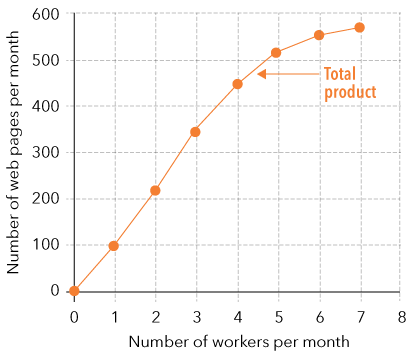Figure 7.3: Total production as a function of number of workers​

The most obvious conclusion drawn by looking at Figure 7.3 is that the production function is upward sloping. An increase in labor causes an increase in total product. But you should notice one other characteristic of a typical short-run production function. The slope of the production function in Figure 7.3 becomes less steep as more workers are added.

As the variable input, labor, increases, output does increase, but the increases in output seem to be getting smaller as more labor is added.

The production function presented here assumes that the amount of labor can vary, but that the amount of capital Patricia has, the technology she is able to use, and how effective the labor is at producing web pages are all fixed. Changes in these normally take longer to occur than changes in the amount of labor hired. They are what we describe as long-run changes. The long run is a period of time that is long enough for all inputs to be changed. To make sure we understand what a production function actually means, let’s see how the production changes if those other inputs do change.

### Graphing Question 7.02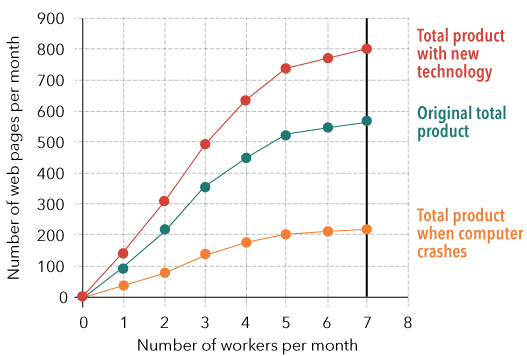Figure 7.4: With new technology, the total product function shifts upward. At each level of input, the firm is able to produce more. With fewer computers to use, each worker is able to produce less and the total product function shifts down.​

Question 7.01

Match the input to its correct category.

Premise
Response
1

Laptop computer

A

Capital

2

Salmon

B

Capital

3

Janitor

C

Labor

4

Accountant

D

Land

5

Corner Lot

E

Labor

6

Office building

F

Land

Question 7.02

A production function can best be described as which of the following?

A

A graphical depiction of what can and cannot be produced with a given amount of inputs

B

The quantity of inputs required to produce each unit of output in a given amount of time

C

The relationship between the quantity of inputs and quantity of outputs produced in a given amount of time

D

The quantity of outputs created by a given quantity of inputs in a given amount of time

### Question 7.03

Question 7.03

In economics, we introduce simple models that assume inputs are identical. That is, all workers are exactly the same. Is this a realistic assumption? How might it be harder to focus on specific aspects of models if we are worried about the different talents that workers bring to the production process?

Hover here to see the hint for Question 7.03.

## 7.3 Marginal Product

Remember our very first chapter discussion of the importance of thinking at the margin? To economists, this means evaluating the effects of small adjustments or additions to existing situations. The marginal product of labor is the change in the amount of output that results from a change in the amount of labor employed while holding other inputs constant. In Table 7.2, we can see the additional web pages designed for each additional computer programmer hired. The marginal product of the first worker is the increased production that worker adds. In this case, that is 100 web pages. The marginal product of the second worker is the change in output that results from adding one more worker, that is, 220 web pages minus 100 web pages or 120 web pages. The calculations are graphed in Figure 7.4. Notice that the marginal product increases as we add the second and third workers, but it begins to decrease with the fourth worker and continues to diminish after that.

In Table 7.2, the marginal product for the first worker is listed between the rows for zero workers and the first worker. The marginal product for the second worker is on a line between the rows for the first and second worker. We use that mechanism throughout the table. The reason is to emphasize that it is the change in total product that results from adding each worker. The same format is used for graphing marginal product. Each marginal product is plotted at the midpoint between each number of workers. To represent marginal product algebraically, let MP represent the marginal product, Δ TP represent the change in total product when the number of workers changes, and ΔL represent the change in the number of workers (Δ is the Greek letter delta and is used in math to represent the phrase “change in”). The resulting equation is:

The law of diminishing marginal returns. Given that some resources are fixed (in the case of Patty’s Pages, capital, in addition to technology and the quality of labor), there is a special relationship between total product and the amount of labor hired. As more labor is used, total product will increase, as shown in Figure 7.3. Initially, the increases may get larger. Two workers may provide an opportunity for specialization, where they perform different tasks that help each other be more productive. In contrast, one worker would have to produce both tasks, perhaps switching back and forth to get the job done. However, the increases in total product from each additional laborer will eventually get smaller, as shown in Figure 7.5. That is the law of diminishing marginal returns. (The law of diminishing returns is referred to in the popular press as simply diminishing returns, and sometimes in economic theory as the law of eventually diminishing marginal returns or diminishing marginal product.)

The logic of the law of diminishing marginal returns is that as the additional labor has less of the fixed factor with which to work, the additional worker will not be able to produce as much as the initial workers hired. Assume that there are three computer workstations in our web page development office. Three web page programmers can use the computers all day or at least use them at will.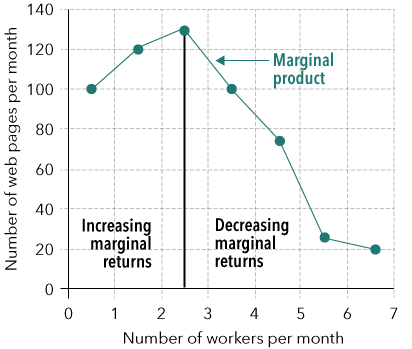Figure 7.5: Marginal product as a function of the number of workers​

With a fourth worker, at least two must share the computers. Each will be able to use the computer for only part of the day. Therefore, that fourth worker is not likely to be able to add as much to production as each of the first three workers.

The marginal product can diminish as soon as an employer begins to add workers. It is more common that adding workers will increase marginal product initially. The addition of a second worker may allow both the first and the second worker to specialize, learn their own jobs better, and produce more than twice what one worker can. If this is the case, the firm will experience an increasing marginal product. And that is what we see in our example. The marginal product of the second worker is higher than the first, and the marginal product of the third is higher than the second.

Eventually, the increase in the marginal product will end, and the marginal product will begin to diminish. The fact that marginal product may increase as an input is increased and then begin to decrease as more is added is why economists refer to this phenomenon as the law of eventually diminishing returns. It is even conceivable that we could use so many workers that they begin to interfere with one another and total product could decrease as we add workers (a negative marginal product). It is important to note that in this simple model, we assume all workers have the same basic skills. The changes we observe in marginal productivity are simply a function of how many workers we hire. Of course, in the real world, an employer would like to hire the most productive workers first.

You may have witnessed a moment of diminishing marginal productivity becoming negative: When a new fast food restaurant opens, it must train its new workers who often have little or no previous experience. One way to accomplish the training is to have all the new workers show up on the same day and work together. Customers will see more workers than normal. But rather than getting their food faster, customers might experience delays. All of the trainees, rather than speeding up service, may actually be getting in each other’s way. After all, there is only so much room, only so many cash registers, and only so many fryers to work with.

But we will seldom observe a profit-maximizing firm hiring so much labor that production actually falls. Only if the firm is making a mistake will that result occur. If the firm’s goal is to maximize profits, a decision to increase costs and reduce output at the same time makes no sense.

You can also see evidence of diminishing marginal returns to labor by looking at the slope of the total product curve. The slope is the change in total product divided by the change in the amount of labor, and that is the same as our definition of marginal product. The slope increases as the firm expands from no labor to three workers. With even more labor, the slope begins to decrease.

With fewer than three workers, we are experiencing increasing marginal returns to labor. At three workers, we reach the point of diminishing marginal product. Thereafter, the slopes, of the total product curve and the marginal product of labor decrease.

Question 7.04

Marcus has four employees. The four employees produce 55 floral arrangements in a day. Marcus hires a fifth employee. The five employees produce 60 floral arrangements in a day. The fifth employee’s marginal product is __________.

A

60 floral arrangements in a day

B

5 floral arrangements in a day

C

11 ¾ floral arrangements in a day

D

12 floral arrangements in a day

Question 7.05

What does diminishing marginal productivity mean?

A

As you increase the amount of a variable input, its average product eventually gets smaller.

B

As you increase the amount of a variable input, its marginal product eventually gets smaller.

C

As you increase the amount of a fixed input, its marginal product eventually gets smaller.

D

As you increase the amount of a variable input, total output eventually declines.

### Question 7.06

Question 7.06

If every worker brought into a production process is identical, why does marginal productivity generally decrease?

Hover here to see the hint for Question 7.06.

## 7.4 Average Product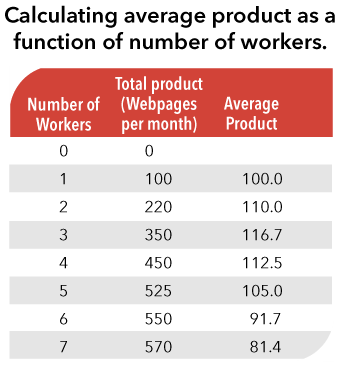Table 7.3: Calculating average product as a function of number of workers​

A third concept rounds out the picture of production. The average product of labor is the amount of total output produced divided by the amount of labor hired holding all other inputs at the same level. It is what each worker produces on average. In our example, the average product of labor with one worker is total product, 100 web pages, divided by one worker. Average product is equal to 110 web pages per worker (220 web pages / 2 workers) when two workers are hired and 116.7 web pages per worker (350 web pages / 3 workers) when three workers are hired. The calculations are shown in Table 7.3 and graphed in Figure 7.6. Average product is the productivity variable that news stories often refer to in discussing sources of growth in companies or in the entire economy. But we will see that while average product is important, marginal product is even more important for decision-making.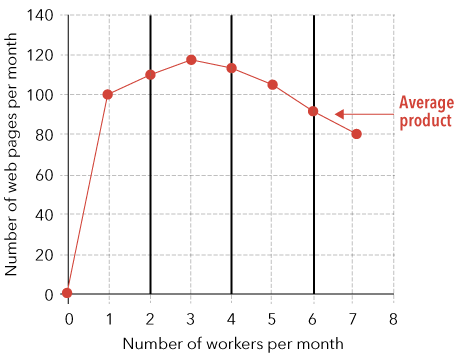Figure 7.6: Average product is the total product divided by the amount of the variable input. This relationship shows an increasing average product as more labor is used, followed by falling average product with higher amounts of labor.​
Question 7.07

The production of 12,000 candy bars per day requires 60 workers. The average product of each worker is ______________ candy bars per day.

A

12,000

B

600

C

200

D

20

Question 7.08

If the average product of labor is seven units of output per worker per day, the total output of 15 workers will be ______________ units per day.

A

105

B

7

C

2 1/7

D

70

### Question 7.09

Question 7.09

In economics, we often focus on the margin of a problem, so the presentation of marginal product comes before the presentation of average product. Why do you think we want you to learn about the average product of labor?

Hover here to see the hint for Question 7.09.

## 7.5 The Relationship Between Marginal Product and Average Product

When we put marginal and average product together on a single graph, one important point emerges – marginal product draws average product toward itself. In this example, when marginal product is above average product (from zero to three workers per week), average product rises toward marginal product. When marginal product drops below average product (with more than three workers), average product turns and starts declining. In fact, when marginal product is exactly equal to average product, average product is at its maximum point.

### Question 7.10

Question 7.10

Look at Figure 7.7 below. Describe the relationship between the marginal product and average product functions.

Hover here to see the hint for Question 7.10.

An example might help. Think of a group of three individuals, all of whom are 1.8 meters tall. The average height of individuals in the group is 1.8 meters [(1.8 + 1.8 + 1.8) / 3 = 1.8 meters]. Suppose we add a fourth individual to that group and that individual is 2.0 meters tall. What will happen to the average height of individuals in the group?

Because we are adding a taller person to the group, the average will increase (to 1.85 meters). [(1.8 + 1.8 + 1.8 + 2.0) / 4 = 1.85 meters]. If that fourth person were 1.6 meters tall, the average of the group would fall (to 1.75 meters) [(1.8 + 1.8 + 1.8 + 1.6) / 4 = 1.75 meters]. What happens to the average depends upon the size of the additional person relative to the height of those already in the group.

We can apply the same logic to our model. If we add a worker whose additional, that is, marginal, contribution is greater than the average of all the past workers, then the average contribution of all the workers must increase. If the contribution of the additional worker is less than the average of the previous workers, the overall average will fall. The importance is that when a more productive worker is hired, average product rises.  Conversely, when a less productive worker is hired, average product falls.Figure 7.7: Average and marginal products are shown together. Notice the interaction. If marginal product is greater than the average product, the average product will be rising. That is true regardless of the direction of change of marginal product.​
Question 7.11

Consider two students, each earning 1300 on the quantitative and verbal portions of the SAT. The average SAT score for our group of two is 1300, of course. (1300 + 1300)/2. Suppose we add one more student to the group and calculate the new average. What will the new average be if the third student has an SAT that is equal to 1300?

Question 7.12

What is the average score if the third student has an SAT score equal to 1000?

Question 7.13

What is the average score if the third student has an SAT score equal to 1600?

### Question 7.14

Question 7.14

In one case the average went up, and in the other case the average went down. Explain why.

Hover here to see the hint for Question 7.14.

Question 7.15

Currently, the marginal product of labor is 45 units per week. The average product of labor at the current level of output is 32 units per week. If the employer hires one more worker, the marginal product of labor will be 47 units per week. The average product of labor will ______________.

A

Equal the marginal product of labor

B

Fall

C

Rise

D

Stay the same

Question 7.16

Currently, Frank has 10 employees. The marginal product of the 10$^{th}$ worker is 25 units per week. The average product of the 10 workers is 20 units per week. If Frank hires one more worker, the marginal product of the 11$^{th}$ worker will be 24 units per week. The average product of labor will ______________.

A

Stay the same

B

Rise

C

Fall

D

Equal the marginal product of labor

Question 7.17

Fill in the missing value for A in the table below.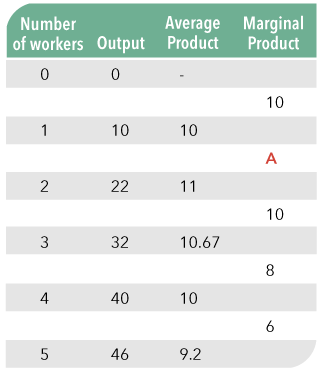A

10

B

11

C

12

D

22

Question 7.18

Fill in the missing value for B in the table below.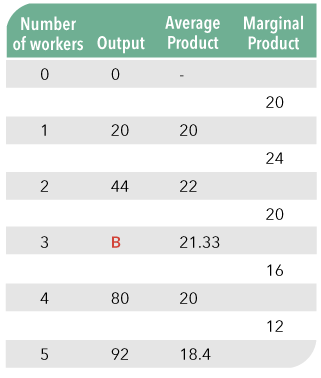A

64

B

62

C

66

D

60

Question 7.19

Fill in the missing value for C in the table below.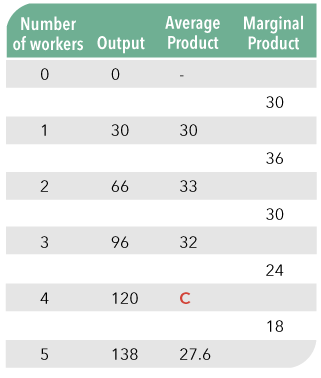A

36

B

33

C

24

D

30

### Question 7.20

Question 7.20

Describe how you calculated the values for A, B, and C in questions 7.17 – 7.19.

Hover here to see the hint for Question 7.20.

### Question 7.21

Question 7.21

While marginal productivity pulls the average productivity function toward itself, why does the strength of the influence of marginal product weaken as output rises?

Hover here to see the hint for Question 7.21.

Question 7.22

Diminishing marginal returns means that marginal product will eventually ______________ and the total product function will ______________ as production increases.

A

Increase; not change

B

Decrease; not change

C

Increase; decrease

D

Decrease; decrease

E

Not change; not change

Question 7.23

The slope of a firm’s production function will ______ as the amount of a variable input used increases if the input experiences diminishing marginal productivity.

A

Not change

B

Be indeterminate depending on the amount of the variable input used

C

Increase

D

Decrease

## 7.6 Costs and Outputs

A profit-maximizing producer does not just look at potential revenues or the number of workers needed to produce each level of output when deciding how much to produce. To estimate potential profits, the producer must calculate costs. The producer will want to know the costs of the workers and other resources used. That is why we add the prices of the inputs to our model of the firm. By doing so, we will be able to calculate the total cost at each level of output, the average cost of the product, and the cost of expanding output. In the process, we should be able to describe the costs of Patty’s Pages.

### 7.6.1 Fixed Costs

What is the cost of capital? There are three common ways to consider how business owners pay for capital: One way is to buy the capital outright. But it still has a cost every period. The cost of owning the capital is the investment income the money could have earned. A second way is to borrow money to buy the capital. With this approach, the cost is the interest paid each time period. The third way is to lease the capital. With this approach, the business owner pays the lease price. In all three ways, economists refer to the price of capital as the rental rate of capital. This should not be confused with the fact that all capital will wear out or depreciate over time.

Remember that we began this chapter by assuming that some inputs are fixed. Before considering how much labor to hire, Patricia of Patty’s Pages had rented office space and purchased some computer workstations. Those fixed resources have a cost. For each fixed resource, we multiply the price of the fixed resource times the number of resources used. We add the results, and the sum is the total fixed cost. It is fixed in the sense that as output varies, that part of total costs does not change. In fact, this is the only cost when total product is zero, and it is the same when output is much higher. The reason fixed costs remain even if output is zero is that the producer is still obligated to make payments on these inputs no matter what the level of output is.

In our web page example, the total fixed cost is the cost of three computer workstations, office space, and some supplies—a total fixed cost we will assume is $5,000 per month. Table 7.4 shows the total fixed cost for producing web pages with one to seven workers. By its nature, total fixed cost does not change as the number of workers hired increases and as total product increases. Total fixed cost and the corresponding levels of output are graphed in Figure 7.8. Note that, now that we are looking at costs relative to the level of output, our Y axis will be measured in dollars, and our X axis will be measured in units of output. The horizontal line emphasizes that unchanging nature of the fixed costs.Table 7.4: Fixed cost remains the same for all levels of output.​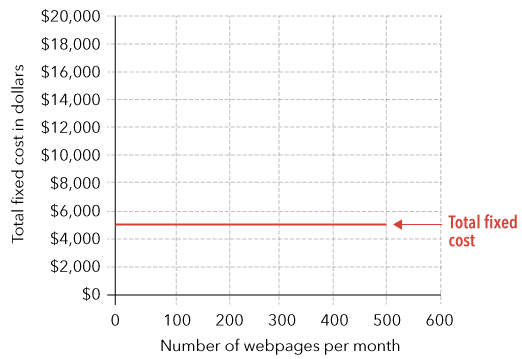Figure 7.8: Total fixed cost is equal to$5,000 at all levels of output. An increase in any part of fixed cost will shift the total fixed cost curve upward. A decrease will shift the curve down.​

Question 7.24

If the quantity of an input is fixed in the short run, its total cost will ______________ as output increases.

A

Increase

B

Decrease

C

Stay the same

D

Rise then fall

### Question 7.25

Question 7.25

If a fixed cost does not change as output changes, why do you still have to pay it if you produce zero output?

Hover here to see the hint for Question 7.25.

### 7.6.2 Variable Costs

Business owners increase and decrease production by adding and subtracting variable inputs. In the case of Patty’s Pages, our web page design firm, there is only one variable input: labor. The amount of the variable input used times its price is the total variable cost. The cost is variable because the cost changes as we expand production by adding more of the variable input. If there were more than one variable input, the costs of each will be added to get the total variable cost. In column four of Table 7.5, the total variable cost corresponding to each number of workers hired is calculated. We will assume that the going monthly wage for web page developers is $3,000 per month. With zero workers, the total variable cost is zero; with one worker,$3,000; with two workers, $6,000, and so forth.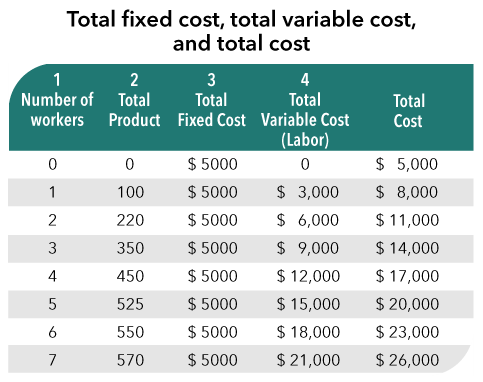Table 7.5: Total fixed cost, total variable cost, and total cost​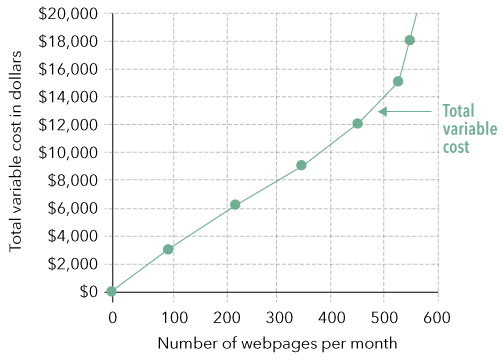Figure 7.9: Total variable cost as a function of output​ We graph total variable cost at each level of output in Figure 7.9. In contrast to total fixed cost in Figure 7.8, total variable costs do increase as production increases. To simplify our model, we assume there is just one wage. An employer has no ability to pay any wage less than the assumed wage because other employers will employ the workers she needs if she offers anything less than the going wage. But she also has no incentive to offer more as long as workers will come and work for her at this wage. Therefore, variable cost is simply the assumed wage rate per time period times the number of workers. Question 7.26 If the quantity of an input is variable in the short run, its total cost will ______________ as output increases. A Increase B Decrease C Stay the same D Rise then fall Question 7.27 If labor is the only variable input a firm owner uses and the wage rate is$200 per week, what is the firm owner’s variable cost per week if she hires 12 workers?

A

$200 B$1,200

C

$2,400 D It depends on the level of output. ### Question 7.28 Question 7.28 If a store owner is doing her best to minimize cost, why is it still true that total variable cost has to increase when output increases? Hover here to see the hint for Question 7.28. Click here to see the answer to Question 7.28. ### 7.6.3 Total Cost Total variable cost plus total fixed cost at each level of output is equal to the total cost of production at each level of output. The two costs are added together in Table 7.5 for each number of workers and in Figure 7.10 for each level of output. Because total fixed cost is constant at each output and variable cost increases as output increases, the total of the two will increase as output increases. At a total product of 220, total cost equals$11,000 ($5,000 +$6,000). At a total product of 350, total cost equals $14,000 ($5,000 + $9,000).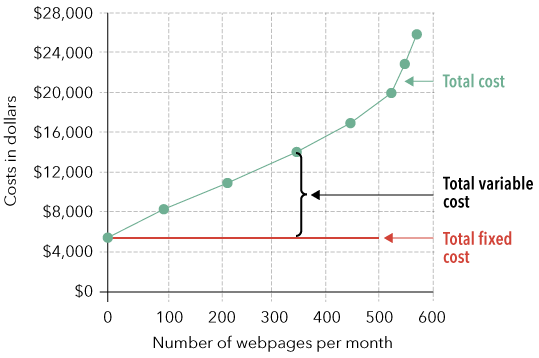Figure 7.10: Total cost as a function of output​ Question 7.29 If at 500 units of output, total fixed cost is equal to$10,000 and total variable cost is equal to $15,000. Total cost is equal to _____. A$50

B

$15,000 C$25,000

D

$1,250,000 Question 7.30 At 1,000 units of output, the fixed cost of production is$12,500 per week. Total cost of producing 1,000 units per week is $28,500 per week. The variable cost of producing 1,000 units of output per week is equal to _____. A$16,000

B

$41,000 C$16

D

$41,000,000 ### Question 7.31 Question 7.31 How do we consider total cost for a given period of time if some costs are fixed and some costs are variable? Hover here to see the hint for Question 7.31. Click here to see the answer to Question 7.31. ### 7.6.4 Marginal Cost While total cost is important, to make a decision about increasing or decreasing output, a producer really wants to know the rate at which total cost changes as output changes. And that is marginal cost – the cost of producing one more unit of output, or in our example, developing one more new web page. When we add the first worker to our company, the marginal cost is the increase in total cost ($8,000 - $5,000) divided by the increase in output (100 new web pages designed). It is equal to$30 per additional web page designed. Total cost increases by $30 for each new web page designed when we add one worker. If Patricia expands from hiring one to two workers, the marginal cost changes to$25 per web page. [($11,000 -$8,000) / (220 web pages – 100 web pages) = $25 per additional web page designed] The results are shown in Table 7.6 and graphed in Figure 7.11.Table 7.6: Total fixed cost, total variable cost, and total cost​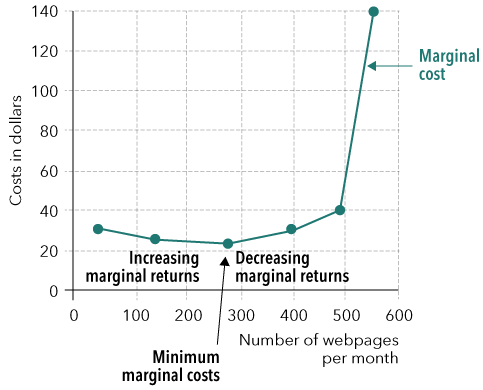Figure 7.11: Marginal costs as a function of output​ Marginal cost is listed in Table 7.6 between each number of workers, representing that the marginal cost is the increase in cost the firm experiences from increasing the quantity of output from one level to the next. The marginal cost is plotted in Figure 7.11 at the midpoint between the quantities produced. Question 7.32 Consider this example: The total cost of producing 1,000 units of output is equal to$55,000 per week. The total cost of producing 1,010 units is equal to $55,500 per week. The marginal cost of increasing output from 1,000 units per week to 1,010 units per week is: A$500

B

$50 C$10

D

$5 Question 7.33 Alicia is currently spending$6,000 per week on total variable costs to produce 500 hats. To produce 505 hats per week, she would have to spend $6,100 per week. The marginal cost per hat is ______. A$6,100

B

$100 C$20

D

$5 ### Question 7.34 Question 7.34 If workers become more productive as more are hired, explain what happens to the value of labor to a producer? Hover here to see the hint for Question 7.34. Click here to see the answer to Question 7.34. ### 7.6.5 Diminishing Marginal Returns Causes Increasing Marginal Cost Notice that as total product increases, marginal cost decreases slightly at first and then begins to increase. The changes in marginal cost appear to be the opposite of the changes we saw in the marginal product. When we calculated marginal product, we found that the marginal product increased at the beginning as more labor was hired. Eventually, marginal product began to decrease. One way to see this is to look at graphs of marginal product of labor and marginal cost side-by-side (Figure 7.12)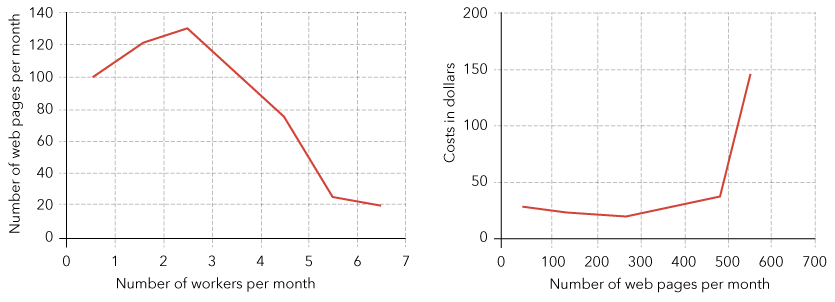Figure 7.12: Marginal product of labor vs. marginal cost.​​ When marginal product is increasing, marginal cost is decreasing. The logic behind the observation is that it takes less additional labor to increase output if labor gets more productive. Therefore, it takes a smaller increase in variable costs to increase output. However, when marginal product starts to decline, an employer must hire larger increments of labor to expand output. As a result, marginal cost starts to increase. If marginal product is decreasing as the number of workers is increased, it must be that each additional worker is adding smaller amounts of output. Given that each additional worker costs the firm the same amount, the cost of those additional units of output, the marginal cost must be increasing. The third worker added to Patty’s Pages costs$3,000 a month and develops an additional 130 web pages. The fourth worker also costs $3,000 and adds 100 web pages to total product. Marginal product declines from 130 to 100 web pages per worker. Marginal cost increases because the$3,000 cost is spread across fewer web pages. When Patricia adds the third worker, marginal cost is $3,000/130 web pages =$23 per web page. The marginal cost when adding the fourth worker is $3,000/100 web pages =$30 per web page.

An alternative explanation is an algebraic one. Marginal cost is equal to the change in total cost divided by the change in total product. The change in total cost equals the change in the number of workers times the wage rate. Thus, marginal cost is equal to the wage rate times the change in the number of workers divided by the change in total product. If marginal cost is MC; total cost is TC; total product, TP; the number of workers or the amount of labor, L; the wage rate, W; and ‘the change in’ is Δ, then:

Recall from earlier in the chapter that the marginal product (MP) is equal to the change in total product divided by the change in number of workers.

Marginal cost, then, is the inverse of marginal product times the wage rate. Given that wages are fixed, when marginal product increases, marginal cost must decrease. When marginal product falls, marginal cost must rise.

### Question 7.35

Question 7.35

Assume that wages are $20 per hour; at the current number of hours of labor employed, the marginal product of an hour of labor is 10 units of output. Labor is the only variable input. What will happen to marginal cost if you hire one more hour of labor and the marginal product of the next hour of labor employed increases to 15 units of output? Hover here to see the hint for Question 7.35. Click here to see the answer to Question 7.35. Question 7.36 Using the information from the table below, what is the marginal cost of increasing output from 32 to 40 knit scarves per week if the weekly wage is$200?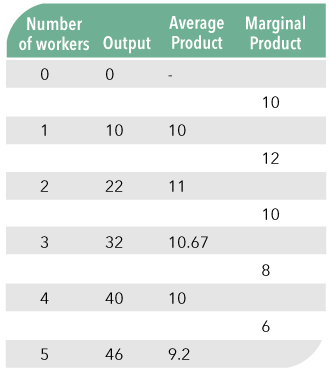A

$50 B$25

C

$200 D$4

Question 7.37

Use the information from the table. If the weekly wage is $200, the marginal cost of increasing weekly production from 10 to 22 scarves per week is ______________ than the marginal cost of increasing weekly production from 40 to 46 scarves per week because the marginal product of the second worker is ______________ than the fifth worker.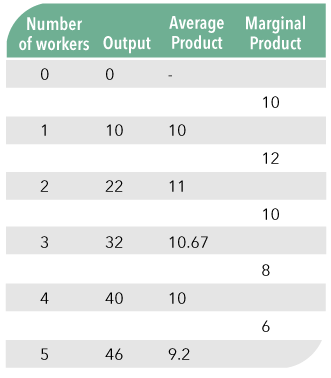A Higher, higher B Lower, lower C Higher, lower D Lower, higher ### Question 7.38 Question 7.38 Why is increasing marginal cost a short-run phenomenon? Hover here to see the hint for Question 7.38. Click here to see the answer to Question 7.38. ### 7.6.6 Average Cost Our final new cost concept is average cost. Average cost is the total cost at each level of output divided by the total product. Along with total cost, it is a concept often included in news stories. In Table 7.7, average cost for Patty’s Pages, the web page development firm, is calculated. Average cost is graphed and shown in Figure 7.13, along with the marginal cost function. The table and the graph show that at low levels of output, an expansion of output causes average cost to fall. At an output level of 450 web pages a month, average cost reaches a minimum. When output is increased beyond 450 web pages, average cost increases.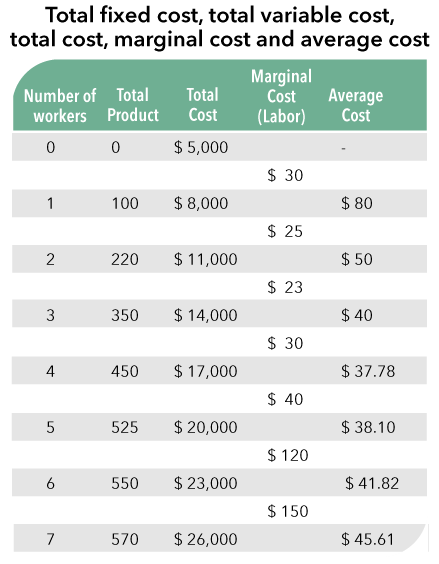Table 7.7: Total fixed cost, total variable cost, total cost, marginal cost, and average cost​Figure 7.13: Average and marginal costs as a function of output​ The reasons for this u-shaped average cost curve are based on the relative sizes of total fixed and total variable costs. Total fixed cost is a large portion of total cost at low levels of output. In our example, when 100 web pages are produced, total fixed cost is$5,000 and total variable cost is $3,000. The primary reason that average cost falls as output rises is that the fixed cost is spread out over more web pages. What was once a large part of average cost becomes smaller as the quantity output increases. As production of web pages continues to increase, more total variable costs are incurred. Because of diminishing returns, those total variable costs increase at a faster rate as output continues to expand. The total variable cost eventually becomes the largest portion of total costs. For example, at a production level of 550 web pages, fixed cost is$5,000 and variable cost is $18,000. The increases in variable cost per unit of output eventually outweigh the decreases in fixed cost per unit of output. When that happens, average costs begin to rise. Question 7.39 What are two of the reasons that average cost tends to have a “bowl” shape? A Fixed costs tend to dominate high levels of output and variable costs tend to dominate low levels of output B Fixed costs tend to dominate low levels of output and variable costs tend to dominate high levels of output C Fixed costs and variable costs tend to dominate low levels of output D Fixed costs and variable costs tend to dominate high levels of output Question 7.40 The fixed cost of producing wedding cakes is$10,000 per month. The variable cost for producing 10 wedding cakes per month is $12,000. The average cost of producing 10 wedding cakes per month is ____. A$1,000

B

$1,200 C$2,200

D

### Question 7.42

Question 7.42

Describe what happens to the average cost of production at Patty’s Pages when Patricia increases output from 525 web pages per month to 550 web pages per month.

Hover here to see the hint for Question 7.42.

### Question 7.43

Question 7.43

Explain the relative position of a general average cost curve with respect to its corresponding marginal cost curve from low levels of output to higher levels of output.

Hover here to see the hint for Question 7.43.

Interpret the effects of each of the following for Patricia’s web page development firm:

Question 7.44

Working from the table, assume that instead of the cost of the three computer workstations and space being $5,000 per month, it is$10,000 per month. Match the number of workers to the correct total cost.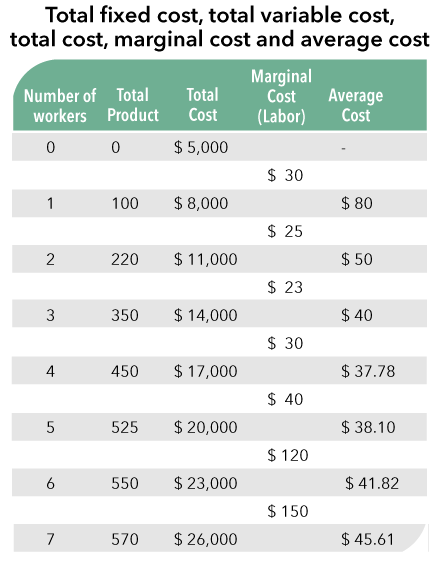Premise
Response
1

0

A

$22¸000 2 1 B$10¸000

3

2

C

$31¸000 4 3 D$16¸000

5

4

E

$25¸000 6 5 F$19¸000

7

6

G

$13¸000 8 7 H$28¸000

Question 7.45Working from the table, assume that instead of the cost of the three computer workstations and space being $5,000 per month, it is$10,000 per month. Match the number of workers to the correct marginal cost.

Premise
Response
1

0

A

$25 2 1 B$30

3

2

C

$40 4 3 D$23

5

4

E

0

6

5

F

$30 7 6 G$150

8

7

H

$120 Question 7.46 Assume that instead of the cost of the three computer workstations and space being$5,000 per month, it is $10,000 per month. Match the number of workers to the correct marginal cost.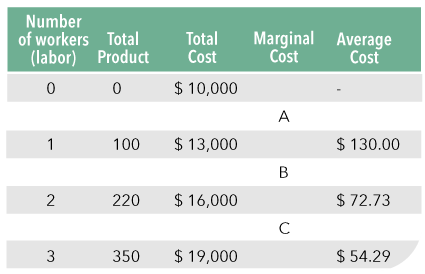A A =$3,000, B = $3,000, C =$3,000

B

A = $3,000, B =$1,500, C = $1,000 C A =$30.00, B = $25, C =$23.08

D

A = $30.00, B =$13.64, C = $8.57 To get a better understanding of how costs behave, we will analyze what happens to when each of the following changes occur: fixed costs increase, wages increase, and productivity increases. Each change is presented separately in the following three tables. The change in fixed cost in Table 7.8 changes average and total cost. Nothing in this problem has impacted the marginal product of additional units of labor hired. Therefore, it does not change marginal cost, as marginal cost is the change in total cost that results from a change in output.​​Table 7.8: Total fixed cost, total variable cost, total cost, marginal cost, and average cost The change in wage rates is a change in variable costs. Total costs are affected here. Thus, total cost and average cost decrease. Marginal cost decreases as the additional resources now cost less. This is reflected in Table 7.9.​Table 7.9: Total fixed cost, total variable cost, total cost, marginal cost, and average cost​​ The change in the production function means that marginal and average product increase. Thus marginal and average cost will decrease. Total cost at each level of output also decreases. This is reflected in Table 7.10.​Table 7.10: Total fixed cost, total variable cost, total cost, marginal cost, and average cost​​ Question 7.47 At 1,000 units of output, the fixed cost of production is$12,500 per week. Total cost of producing 1,000 units per week is $28,500 per week. If labor is the only variable input and the weekly wage is$1,600, how much labor is being used produce 1,000 units of output?

A

5.0

B

17.8

C

16.0

D

10.0

Question 7.48

Juan wants to increase production at his confection shop. If he hires one more worker, he can increase output by 100 candies per week. A confection worker’s weekly wage is $200. Juan’s marginal cost of increasing output by 100 candies per week is ______. A$0.50

B

$1.00 C$1.50

D

$2.00 ### Question 7.49 Question 7.49 Is it possible for marginal cost to be falling and average cost to be rising? Hover here to see the hint for Question 7.49. Click here to see the answer to Question 7.49. ### Graphing Question 7.07 ### Graphing Question 7.08 ## 7.7 Using Cost and Product Analysis In the opening of this chapter, you were asked to think about an assignment as a summer intern. Now you should have the tools to be of service to Patricia as she tries to figure out how many web pages she can develop per month. To see if you are ready, try this exercise: You are now ready to advise Patricia on the impact of different changes to her market on her ability to produce web pages. Throughout this exercise, assume that you are starting from the information given in Table 7.2 and 7.6, with fixed costs equal to$5,000 per month and web page developer’s wages at $3,000 per month. ### Question 7.50 Question 7.50 Explain to Patricia why her marginal cost increases as she increases output beyond 350 web pages per month. Hover here to see the hint for Question 7.50. Click here to see the answer to Question 7.50. ### Question 7.51 Question 7.51 Working from the table above, if Patricia wants to expand output from 450 web pages per month to 525 web pages per month her average cost per web page will go from ______________ to ______________. Hover here to see the hint for Question 7.51. Click here to see the answer to Question 7.51. Question 7.52 Working from the table, if Patricia is currently producing 450 web pages per month. If her landlord increases her rent such that her fixed costs rise from$5,000 per month to $6,000 per month, calculate her new _______.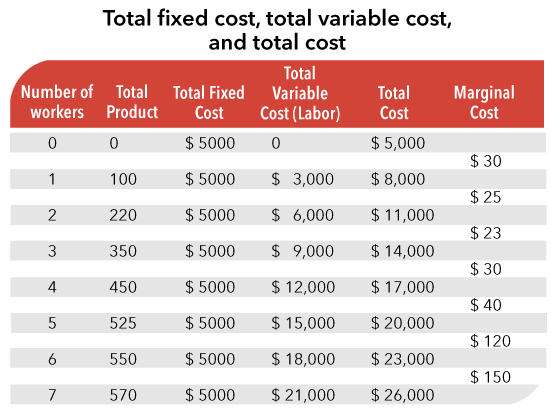Premise Response 1 Variable cost A$40

2

Total cost

B

$30 3 Average cost C$12¸000

4

Marginal cost

D

$18¸000 Question 7.53 Working from the table, if the wage of web page designers increases from$3,000 per month to $3,250 per month. If Patricia produces 450 web pages per month calculate her new _____.Premise Response 1 Variable cost A$32.5

2

Total cost

B

$13¸000 3 Average cost C$40

4

Marginal cost

D

$18¸000 ### Question 7.54 Question 7.54 Assume that web page developers become more productive. Describe how this will impact marginal and average cost at all levels of production. Hover here to see the hint for Question 7.54. Click here to see the answer to Question 7.54. Question 7.55 In the short run, an increase in wages (the price of the variable input) will cause average cost to ______________ and marginal cost to ______________. A Increase; decrease B Not change; not change C Increase; increase D Decrease; decrease E Decrease; increase Question 7.56 In the short run, an increase in the price of one of the fixed inputs will cause average cost to ______________ and marginal cost to ______________. A Increase; decrease B Not change; not change C Increase; increase D Decrease; decrease E Increase; not change Question 7.57 An increase in technology will cause the total product function to ______________ and average costs to ______________. A Increase; decrease B Not change; decrease C Not change; not change D Increase; not change E Increase; increase Question 7.58 When the cost of inputs is constant, diminishing marginal returns means that marginal product will eventually ______ and marginal cost will eventually _______. A Increase; not change B Decrease; not change C Increase; decrease D Decrease; increase ### Graphing Question 7.09 ### Graphing Question 7.10 ## 7.8 Summary • The short run for a firm is a period during which some inputs are fixed. All inputs can be varied in the long run. • Marginal product is the change in total product divided by the change in variable input. Due to the law of diminishing marginal returns, the marginal product of an input will eventually diminish as more of the variable input is used. • When marginal product is greater than the average product, average product will rise. • When marginal product is less than the average product, average product will fall. (Marginal product and average product are equal when average cost is at a minimum.) • Total fixed costs are the costs of the inputs that do not change as output varies. Total variable costs are the costs of the variable inputs at each level of output. Total cost equals total fixed cost plus total variable cost. Total cost increases as output increases. • Marginal cost may decrease as production increases, but will eventually increase as output increases. The increase is the result of the law of diminishing marginal returns. • If, when more of an input is added, marginal product is increasing, then marginal cost will be falling. • If, when more of an input is added, marginal product is decreasing, then marginal cost will be rising • Average cost normally decreases as output increases, but eventually begins to increase. • Average cost decreases when marginal cost is less than average cost. Average cost is at a minimum when marginal cost and average cost are equal. Average cost increases when marginal cost is greater than average cost. ## 7.9 Key Concepts • Production function • Short run • Long run • Total, average, and marginal products • Law of diminishing marginal returns • Total fixed cost, total variable costs, and total costs • Average and marginal costs ## 7.10 Glossary Average cost: Total cost divided by total product. Average product: The total product divided by the number of units of a particular input used. Factors of production: The resources used to produce goods and services, often divided into three categories: labor, all the physical and mental inputs of people; capital, the machines, tools, buildings, and inventories; and land, the actual land used, including raw materials from land. Law of diminishing marginal returns: The marginal product of an input will eventually decrease as more of that input is used. The law of diminishing marginal returns assumes that all other inputs remain constant. Long run: A time period long enough that all inputs can be changed. Marginal cost: The change in total costs that results from increasing total product by one unit (ΔTC/ΔQ). Marginal product: The change in output or product that results from increasing a variable input by one unit while all other variables are held constant. It can be calculated by dividing the change in output by the change in labor used. (ΔTP/ΔL) Production function: A function showing the maximum output for each specific combination of inputs, given technology. Short run: A period in which at least one input or factor of production is fixed. Technological change: A shift in the production function, usually in the direction of a greater quantity of output at each level of input. Technological change may be the result of the creation of new products, the redesign of old products, or the creation of new methods of manufacturing. Total cost: The sum of total fixed cost and total variable cost. Total fixed costs: The costs (prices multiplied by the amounts of inputs) of the inputs that are fixed. This is also the amount of cost when total product is zero. Total fixed costs are costs that do not vary as output changes. Total product: The total amount of output produced. Total variable costs: For a given level of output, the costs (prices multiplied by the amounts of inputs) of the inputs that can be changed. These are the costs that vary as output changes.This Content is Locked Only a limited preview of this text is available. You'll need to sign up to Top Hat, and be a verified professor to have full access to view and teach with the content. ## Answer Keys ### Answer to Question 7.03 The assumption is not realistic. But once we master the ability to look at very general differences (such as between labor input versus capital input), we can look at more specific differences (such as between a managerial worker and a production worker). Click here to return to Question 7.03. ### Answer to Question 7.06 If other inputs are not allowed to increase, workers will eventually have fewer units of capital to work with. Click here to return to Question 7.06. ### Answer to Question 7.09 Yes, marginal product is a very important way to look at the decision to hire one more worker or let one worker go. But average productivity gives us a good way to summarize the overall productivity of our workforce. It allows us to take a step back and look at the bigger picture. Click here to return to Question 7.09. ### Answer to Question 7.10 The average cost function follows the marginal cost function. If marginal cost is less than average cost, average cost falls. If marginal cost is greater than average cost, average cost rises. Click here to return to Question 7.10. ### Answer to Question 7.14 The averages will: a) stay the same at 1300; b) fall to 1200; and c) increase to 1400. If the additional student’s SAT is greater than the average SAT, the average score will increase. If the additional student’s SAT is less than the average score, the average score will decrease. That is the same as is shown in Figure 7.6. If the marginal product is greater than the average product, the average product will increase. Even though the marginal product is declining, as long as it is above the average product, the average product will increase. If the marginal product is less than the average product, the average product will fall. Click here to return to Question 7.14. ### Answer to Question 7.20 To calculate A (MC), you must calculate the change in total output (22-10) and divide it by the change in labor input (2-1) = 12/1 or 12. To calculate B (TP at L = 3), one of two approaches will work. Because AP = TP/L, you could multiply AP ( =21.33) by L (=3) and get 64. Alternatively, you could add MP of the 3rd worker to the previous TP to get 44+20 = 64. To calculate C (AP at L = 4), you divide TP by L = 120/4 = 30. Click here to return to Question 7.20. ### Answer to Question 7.21 Considering your college GPA may help. Early in your college career, each class has a large impact on your GPA because you have so few credit hours. As you have more credit hours, each individual class is a smaller proportion of your overall GPA. Therefore, it is much harder to influence your GPA as a senior than as a freshperson. Click here to return to Question 7.21. ### Answer to Question 7.25 There are some inputs that you must commit to just to get into the market. For example, you may need factory space. You will have to sign a lease or a loan agreement to get access to the space. The owner of the space or the bank that lends you the money does not care how much you produce. They must get paid every month no matter what. You may have to pay out of your own resources if you produce nothing. Click here to return to Question 7.25. ### Answer to Question 7.28 If at every level of output the store owner is minimizing cost, she will never hire any inputs that are not required for any level of output. Therefore, if she wants to produce more output, she will have to hire more inputs which will add to her total variable costs. Click here to return to Question 7.28. ### Answer to Question 7.31 In general, costs of capital are fixed in the short run. All costs are variable in the long run. While it is easy to think of variable costs as having a time component (we hire labor per unit of time), fixed costs are harder to consider as having a time component. Economists think in terms of opportunity costs. If you buy a computer, the money you used to buy the computer can no longer earn interest in some other investment per period of time. Therefore, even a fixed input has a time component. Click here to return to Question 7.31. ### Answer to Question 7.34 If the additional output an employer gets as a result of hiring another worker goes up, the marginal cost of those additional units of output goes down. Consider an increase in marginal productivity from 10 units to 12 units per hour while the wage is$5 per hour. If an employer hires the new worker, the total cost goes up by $5 (the wage). Marginal cost would have been$0.50 under the original marginal product. Now it is $0.42. The labor becomes more valuable to the employer. Click here to return to Question 7.34. ### Answer to Question 7.35 If an hour of labor produces 10 more units of output in an hour and that hour of labor costs$20, the marginal cost is (1 hour x $20) / 10 units of output. That is equal to a marginal cost of$2 per unit. If the next hour of labor’s marginal product increases to 15 units per hour, the  marginal cost will fall to $1.33 per unit. (1 hour x$20) / 15 units of output = $1.33 per additional unit of output. Click here to return to Question 7.35. ### Answer to Question 7.38 To understand this, you need to consider why decreasing marginal productivity is a short-run phenomenon as well. We assumed that marginal productivity decreased as the use of labor increased because we ran out of opportunities for labor to use the capital to produce (there were only so many computer workstations for Patricia’s web page designers to work with). In the long run, we could increase the amount of capital, which would increase the marginal productivity of the workers. As marginal productivity increases, marginal cost falls. It is important to remember that we are assuming all the workers are identical – the observed increase in marginal cost is not because the additional workers are in any way inferior to the ones already hired. They just have less capital to work with. Click here to return to Question 7.38. ### Answer to Question 7.41 Because average costs include fixed costs (average over the level of output), an increase in the underlying fixed costs will shift the average cost curve up and a decrease in the underlying fixed costs will shift the average cost curve down. The marginal cost curve, however, is unaffected by a change in the underlying fixed costs so it will not change. Click here to return to Question 7.41. ### Answer to Question 7.42 To increase output, Patricia must hire one more worker. This worker will cost$3,000. Total output will increase by 25 web pages. Marginal cost will be $120. Because marginal cost is above average cost, average cost will rise from$38.10 to $41.82 per page. Click here to return to Question 7.42. ### Answer to Question 7.43 Generally, the average cost curve will start above the marginal cost curve because you must consider the fixed costs of production as part of the average cost. This means at low levels of output average cost will be relatively large. Marginal cost, however, only examines the change in total cost as output changes. Because fixed costs do not change, they do not impact the marginal cost curve. From that point, marginal cost will pull average cost toward it. As marginal cost rises, it will eventually cross average cost at the minimum point on the average cost curve. From this point on, because the marginal cost curve is above the average cost curve, the average cost curve will rise, giving it its typical bowl shape. Click here to return to Question 7.43. ### Answer to Question 7.49 As long as marginal cost is greater than average cost, average cost will be rising. But if you draw a graph with both marginal cost and average cost (such as in Figure 7.12) you will see that for every point that average cost is rising, not only is marginal cost greater than average cost but it is also rising. What would have to happen for marginal cost to decrease after it has already started to increase is that at some level of production, a new opportunity for increases in marginal productivity would have to appear. With a fixed amount of capital, this is highly unlikely (and well beyond any theory we have developed here). Therefore, we would not expect to witness decreasing marginal cost and increasing average cost at any level of output. (Note: it is possible to see increasing marginal cost and decreasing average cost. Look at the levels of output just before minimum average cost.) Click here to return to Question 7.49. ### Answer to Question 7.50 The marginal product of additional workers declines after the third worker. This means that if she wants the same increase in output, she will have to increase her labor usage by increasing amounts. Or, you can say that she gets smaller increases in output for the same additional cost (the wage of each additional worker). Click here to return to Question 7.50. ### Answer to Question 7.51$37.78 to $38.10. Average cost at output equal to 450 is$17,000/450 and average cost at output equal to 525 = \$20,000/525.

As web page developers become more productive, marginal cost will get smaller at each level of output because the change in output will get bigger for each new worker hired. This will decrease the average cost as well.

### Footnote:

Some text books and on-line sources make a distinction between average variable cost and average total cost. Here, we focus exclusively on average total cost, or simply, average cost.

## Image Credits

 Image courtesy of Peter Tittenberger under CC BY 3.0.

 Image courtesy of Oracle PR under CC BY 2.0.

Factors of production
The resources used to produce goods and services, often divided into three categories: labor, all the physical and mental inputs of people; capital, the machines, tools, buildings, and inventories; and land, the actual land used, including raw materials from land.
Labor
All the physical and mental inputs of people.
Capital
Machines, tools, buildings, and inventories.
Land
The actual land used, including raw materials from land, water, or air.
Production function
A function showing the maximum output for each specific combination of inputs, given current levels of technology.
Short run
A period in which at least one input or factor of production is fixed.
Total product
The total amount of output that can be produced in a given time period with specified amounts of inputs.
Long run
A time period long enough that all inputs can be changed.
Models simplify the real world so that we can focus on and come to an understanding of a few important aspects of what we are studying.
Marginal product
The change in output or product that results from increasing a variable input by one unit while all other variables are held constant. It can be calculated by dividing the change in output by the change in labor used. (ΔTP/ΔL)
Technological change
A shift in the production function, usually in the direction of a greater quantity of output at each level of input. Technological change may be the result of the creation of new products, the redesign of old products, or the creation of new methods of manufacturing.
Law of diminishing marginal returns
The marginal product of an input will eventually decrease as more of that input is used. The law of diminishing marginal returns assumes that all other inputs remain constant.
Consider digging holes with 4 shovels. What would a fifth worker use?
Average product
The total product divided by the number of units of a particular input used.
How do we use averages in everyday situations? For example, why do we care if the average weight in a state or country is rising?
Marginal product draws average product to itself.
Average = sum of observed values divided by the number of observations.
Marginal Product of Labor = ΔTotal Product/ΔLabor, Average Product = Total Product/Labor
Consider what is happening to both the numerator and denominator in the definition of Average Product of labor as output gets larger and larger.
Total fixed cost
The costs (prices multiplied by the amounts of inputs) of the inputs that are fixed. This is also the amount of cost when total product is zero. Total fixed costs are costs that do not vary as output changes.
Consider why and whether or not you pay for variable costs at zero output.
Total variable cost
For a given level of output, the costs (prices multiplied by the amounts of inputs) of the inputs that can be changed. These are the costs that vary as output changes.
If a person is minimizing cost at a level of output, what has to happen to the amount of variable inputs in order to increase output?
Total cost
The sum of total fixed cost and total variable cost.
Total costs are the sum of all the costs that must be paid in the period of time.
Marginal cost
The change in total cost resulting from an increase in production of one unit of output. It can be calculated by dividing the change in total cost by the change in total product.
If workers provide more output per unit of work, what happens to the cost of production?
Recall that there is an inverse relationship between marginal product of labor and marginal cost in the short run.
Consider why marginal cost is rising.
Average cost
Total cost divided by total output.
What does the average cost curve include? What does the marginal cost curve include?
Average cost = total cost/quantity
Work your way from low levels of output to high levels of output (left to right).
MC will draw AC to itself. If MC > AC, AC will rise. If MC